# Classification Metrics for Beginners

## With a function to make it easier to produce and analyze them

With most classification problems a simple accuracy score just will not cut it. Because of this there are many different metrics available to data scientists who are looking to identify how well their model is working. There are benefits and drawbacks with having a plethora of measuring tools at your disposal. The good thing about this is that there is a score for pretty much every problem statement. The bad thing is that there are so many metrics it is often daunting and even confusing to choose them and figure out what they all mean. I will attempt to simplify that situation a bit.

I will detail many of the classification metrics I have come across as a new data scientist. In doing so I hope to define what each metric is measuring and identify a situation in which they would work well.

To get all of the metric scores you must start with calculating the Confusion Matrix. The name of this matrix is aptly named. It can be a bit bewildering sometimes to use it. That being said, it is fundamental to use this in data science. Also, rest assured, once you get the hang of it the confusion dissipates.

Populating the Confusion Matrix is relatively easy and I like to have it charted out for me just for reference. This will be done with Scikit-Learn in a further step but I would like to give a rudimentary example of calculating the matrix first.

If we made 100 predictions on whether or not a coin flip was heads or tails this is how we would calculate the following:

Positive (P): Positive observation. | Heads

Negative (N): Negative observation. | Tails

True Positive (TP): Positive observation that is correctly predicted to be positive.

False Positive/Type I Error (FP): Negative observation that was incorrectly predicted to be positive.

True Negative (TN): Negative observation that was correctly predicted negative.

False Negative/Type II Error (FN): Positive observation that was incorrectly predicted to be negative.

Let’s further our example and actually populate the matrix.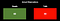Actual Observations for Example Above. This is not a confusion matrix. We will produce the confusion matrix for these numbers below.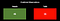Predicted Observations for Example Above. This is not a confusion matrix. We will produce the confusion matrix for these numbers below.

Positive (P): 60

Negative (N): 40

True Positive (TP): 45

False Positive/Type I Error (FP): 0

True Negative (TN): 40

False Negative/Type II Error (FN): 15

These numbers would produce the following Confusion Matrix:

Sklearn’s Confusion Matriximports for both the confusion matrix and the function that plots the confusion matrix. Note: you do not need to run confusion_matrix function in order to run plot_confusion_matrix function. You can just go right to plotting the confusion matrix. Sklearn confusion matrix documentation.

Before you run the Confusion Matrix you need to do all the things you would normally do prior to running a model, run the model and then calculate the models predictions.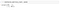Sklearn function that runs just the confusion matrix and returns an array.

To unpack the matrix in order to put it into a Pandas dataframe you must ravel() the matrix first.Here we are doing a few things. We are unpacking the true positives, true negatives, false positives and false negatives and we have also raveled the matrix so that we can unpack these values from the tuples that they are contained in. If we do not ravel you will get a shape of 2 X 2 instead of 4 x 1 and you will not be able to unpack the values from the matrix.

You can also create a DataFrame from the matrix.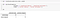Creating a dataframe of confusion matrix values ends up looking a lot like an actual confusion matrix.

Lastly, you can plot the confusion matrix as well.

Accuracy

Accuracy is the most basic metric. It is the percentage of observations (all of the predictions) that were correctly predicted.

Sklearn Accuracy Score

Misclassification Rate

The amount of incorrect predictions among all observations.

Sensitivity/Recall/True Positive Rate (TPR)

This one has a lot of names. Be careful, it can get “confusing.” The ratio of actually positive instances that are correctly predicted to be positive.

Sklearn Recall Score

Precision/Positive Predictive Value

the number of correct predictions among all observations that were predicted positive.

Sklearn Precision Score

Specificity/True Negative Rate (TNR)

How many were correctly predicted among all observations that were actually negative.

F1 Score

Typically when you report precision you also report recall because they are mutually important. So if someone tells you they got a higher precision for example, you should almost always ask them “at what recall?” Conversely, if someone tells you they increased recall then ask them how the precision score was affected. To make this situation simpler, however, there is a score called F1 score. It is the harmonic mean of precision and recall. You’re essentially combining precision and recall into a single metric. The harmonic mean gives much more weight to low values and as a result will only be high if both recall and precision are high.

Note: It is not always necessary to have both metrics scoring at a high level. Sometimes precision is the metric you most care about and at other times recall is the metric that is most important.

Sklearn’s F1-Score

A Series of Functions Producing a DataFrame of Metrics

Below is a function I created to ultimately produce a dataframe including all scores for multiple classifier models. Feel free to use and adapt it. I included a one more scores than what is detailed here. It is called the Balanced Accuracy Score. It can be computed like this in python.

balanced_accuracy = 1/2 * (recall_score + (tn/total_pred))

Step 1. Define your classifiers and compose them into a list.

Step 2. Create the function to instantiate models.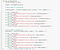Fitting and Scoring the Classifiers on both Train and Test Sets.

The output will look like this:

Step 3. Create Predictions on Test Data and form them into a list.

Step 4. Create Metric Scorer Function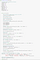Function that creates a dataframe of metrics for a variety of models that you choose. I calculated many of these scores manually using python but you can use the Sklearn functions for some of these as well.

Step 5. Call the function and analyze the output.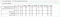Dataframe detailing classification metrics for a variety of models of my choosing. This was ouputted from the custom function created above.

Step 6. I will not detail this step in this article but you will want to interpret the results and produce visuals like ROC AUC curves to further analyze your results.

I hope this helps and you enjoy my custom function. I know there are a million ways to do things and likely simpler ways than what I have created above so if you have tips please message me on medium.

Interested in thoughts and data. Data Science Immersive Student at General Assembly

## More from James Opacich

Interested in thoughts and data. Data Science Immersive Student at General Assembly